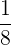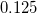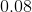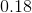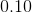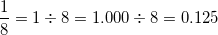GED Math : Numbers

Example Questions

Example Question #6 : Number Lines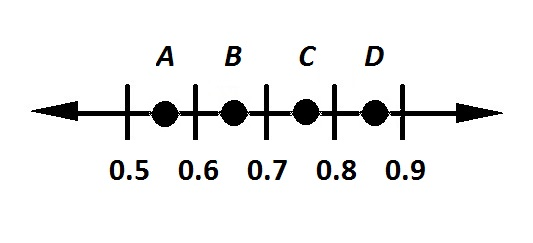Refer to the above number line. Which of the points is most likely the location of the number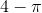?

Do not use a calculator.Explanation: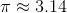, so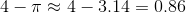.

Therefore,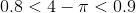.

Of the four points,falls in this range, so it is the correct response.

Example Question #7 : Number Lines

On a number line, how far apart are -2 and 7?

7 units

9 units

-9 units

5 units

2 units

9 units

Explanation:

On a number line, negative numbers lie to the left of zero and positive numbers lie to the right. To count the distance, count the slots between the two numbers: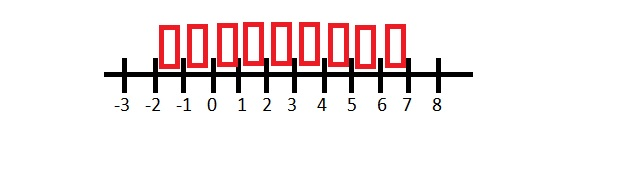There are TWO units between -2 and 0, and there are SEVEN units between 0 and 7. Together, there are NINE units between them.

Example Question #41 : Numbers

On a number line, which of the following is the greatest distance?

The distance between -3 and 0.

The distance between -1 and 2.

The distance between -2 and 2.

The distance between -1 and 2.

The distance between -3 and 0.

The distance between -2 and 2.

All distances are equal.

The distance between -2 and 2.

Explanation:

On a number line, the negative numbers lie to the left of zero and positive numbers lie to the right. To visualize the distance between numbers, look at the units: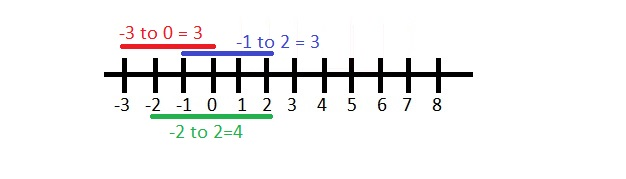-3 and 0 are 3 units apart.

-1 and 2 are 3 units apart

-2 and 2 are 4 units apart. This is the greatest distance.

Example Question #9 : Number Lines

Which distance is greatest?

The distance between -2 and 1.

The distance between 1 and 7.

The distance between -2 and 5.

The distance between -2 and 1.

All distances are equal.

The distance between -2 and 5.

The distance between 1 and 7.

The distance between -2 and 5.

Explanation:

Looking at a number line, you can visualize the distance between positive and negative numbers: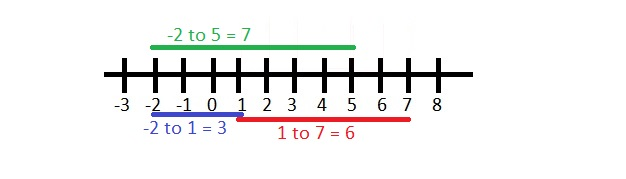There are THREE units between -2 and 1

There are SIX units between 1 and 7.

There are SEVEN units between -2 and 5, so this is the greatest distance.

Example Question #10 : Number Lines

A) The distance between -2 and 5 on a number line.

B) The distance between -1 and 3 on a number line.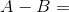4

3

5

6

7

3

Explanation:

The first step is to find out the values of A and B by counting the units between the given numbers.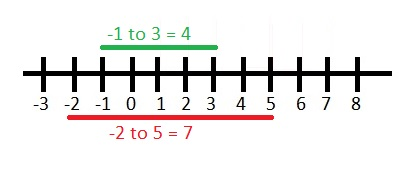A) The distance between -2 and 5 on a number line is 7.

B) The distance between -1 and 3 on a number line is 4.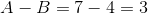Example Question #42 : Numbers

A) The distance between -3 and 1 on a number line.

B) The distance between 2 and 5 on a number line.

C) The distance between -2 and 3 on a number line.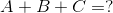8

5

16

12

2

12

Explanation:

To find the distance between any two numbers on a number line, remember that negative numbers lie to the left of zero and positive numbers lie to the right. This is easier to do understand with a picture: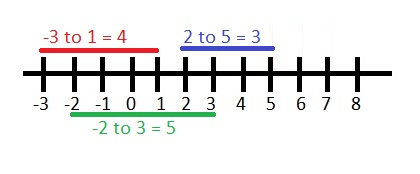A) The distance between -3 and 1 on a number line is 4.

B) The distance between 2 and 5 on a number line is 3.

C) The distance between -2 and 3 on a number line is 5.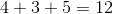Example Question #43 : Numbers

A) The distance between -3 and 0.

B) The distance between -1 and 2.

C) The distance between -2 and 2.11

10

3

5

4

10

Explanation:

To find the sum of these values, you first need to find each individual difference. Use a number line to help visualize the distance between each pair of numbers:A) The distance between -3 and 0 is 3.

B) The distance between -1 and 2 is 3.

C) The distance between -2 and 2 is 4.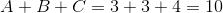Example Question #44 : Numbers

Which of the following number lines represents the inequality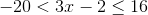?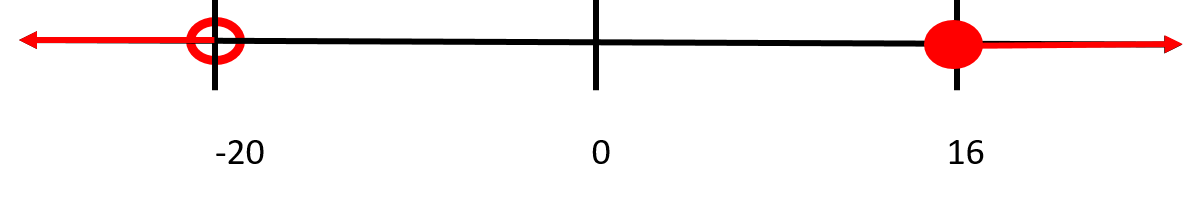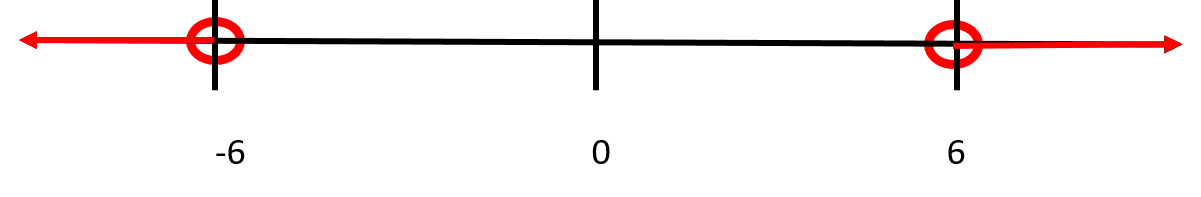Explanation:

Start by solving the inequality.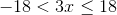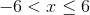Thus, the correct answer should show asbetween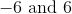.

Recall that a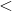sign means that there should be an open circle on the number line, and that a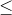sign needs to have a closed circle on the number line.

Example Question #1 : Decimals And Fractions

Convert the following decimal into a fraction: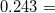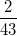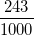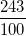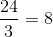Explanation:

The decimal,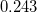, is read as two-hundred forty-three thousandths, which translates to: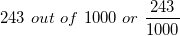Example Question #2 : Decimals And Fractions

Convert the following fraction to a decimal: